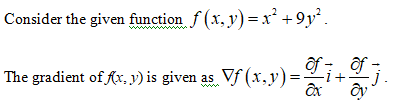# f(x,y)=x^2+9y^2What is the direction in which f changes most rapidly at point (1,2)?

Question
22 views

f(x,y)=x^2+9y^2

What is the direction in which f changes most rapidly at point (1,2)?

check_circle

Step 1Step 2

Gradient of the given function is evaluated as follows.

Step 3

The gradient of the given function ...

### Want to see the full answer?

See Solution

#### Want to see this answer and more?

Solutions are written by subject experts who are available 24/7. Questions are typically answered within 1 hour.*

See Solution
*Response times may vary by subject and question.
Tagged in

### Calculus